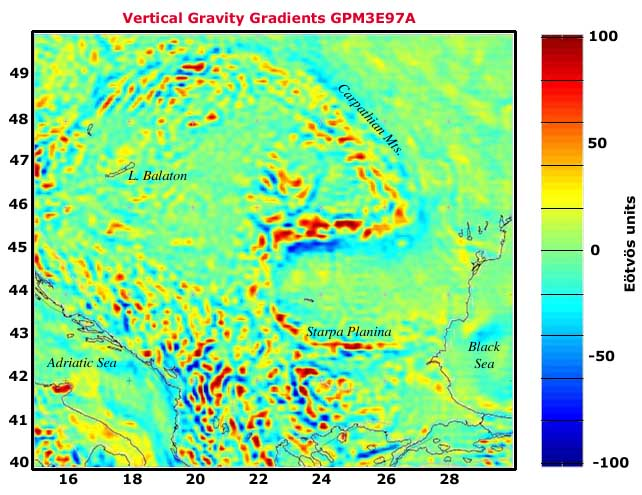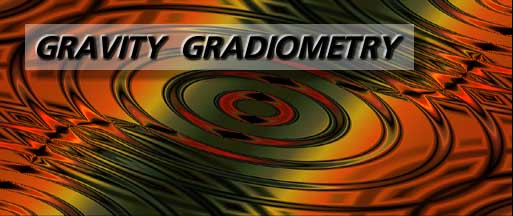Definitions

The gradient of gravity is a quantity that describes the change in gravity through space. The concept becomes a little more abstract, but yet, there is a convenient and intuitive approach to understanding gravity gradients. The material here is from Torge's Gravimetry text, pp. 32-33.

We remember that gravity itself is a vector quantity, g, in three dimensions, given as the triad based on the derivatives of the potential W taken with respect to the three orthogonal directions (here, the consideration is made in Cartesian space). Mathematically, we write: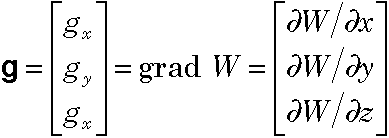The gradient is then a descriptor of how these three components of gravity change in all three dimensions. It is given as a tensor called the gravity gradient tensor or sometimes known as the Eötvös tensor. Mathematically, it is written as: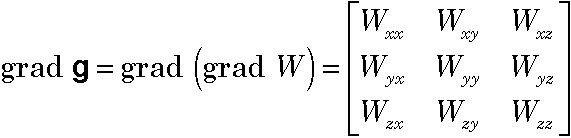where each term of the tensor represents a double partial derivative, e.g.,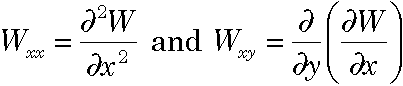The trace of the tensor yields either Poisson's equation (on the surface of the planet) or Laplace's equation (in free space), or, respectively: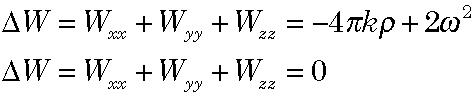Units

Gravity gradients have an interesting physical unit. If we think about this for a minute, what a gradient is, is a change in an acceleration over a span of distance. In otherwords, if we measure gravity in terms of meters per second squared, then a change per unit distance would be "meters per second squared per meter" which would be the same as "per second squared," or "per second per second" which has little intuitive meaning. Furthermore, these quantities are quite small, on the order of ±0.0000001, hence the use of a more convenient unit has been adopted: the Eötvös unit: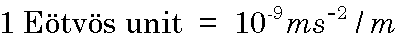Maps

Below is found a sample map of the radial/radial gravity gradient given by the GPM3etz gravity model for Europe: